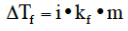# Problem: What is the freezing point of a solution of 9.04 g of I2 in 75.5 g of benzene?(a) Outline the steps necessary to answer the following question.(b) Answer the question.

###### FREE Expert Solution

This would be a freezing point depression problem that can be solved using the equation below.We do not have the molality (m)  to use in the equation so we solve for it first.

87% (175 ratings)###### Problem Details

What is the freezing point of a solution of 9.04 g of I2 in 75.5 g of benzene?

(a) Outline the steps necessary to answer the following question.